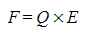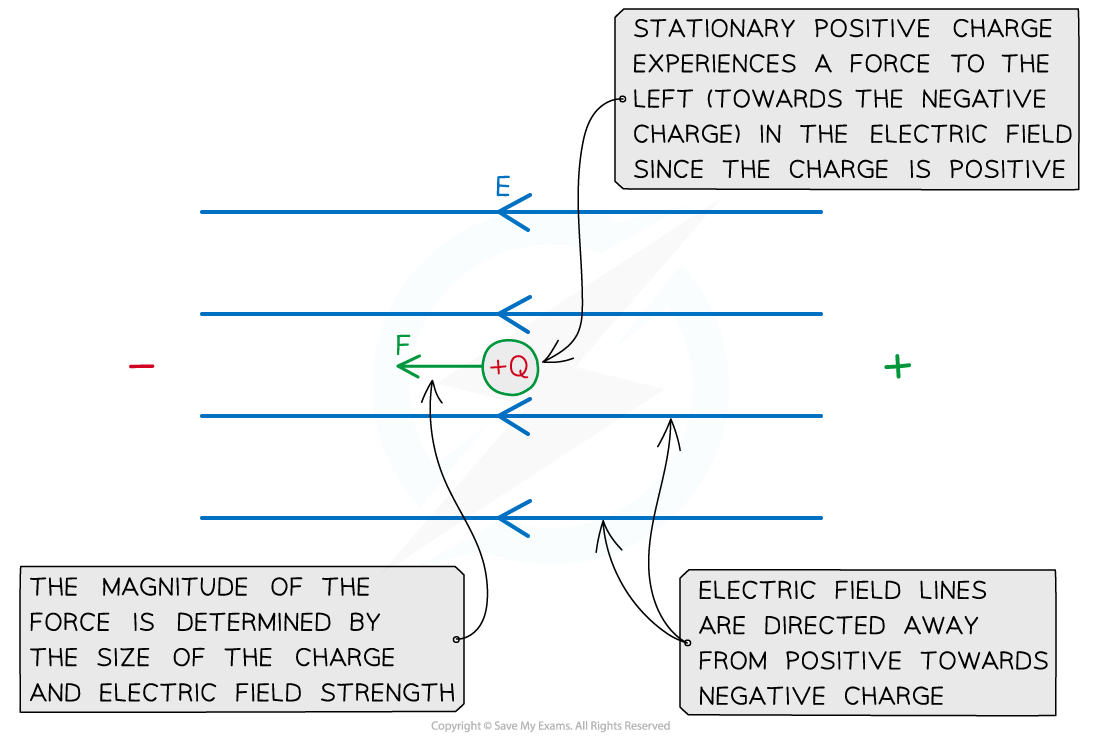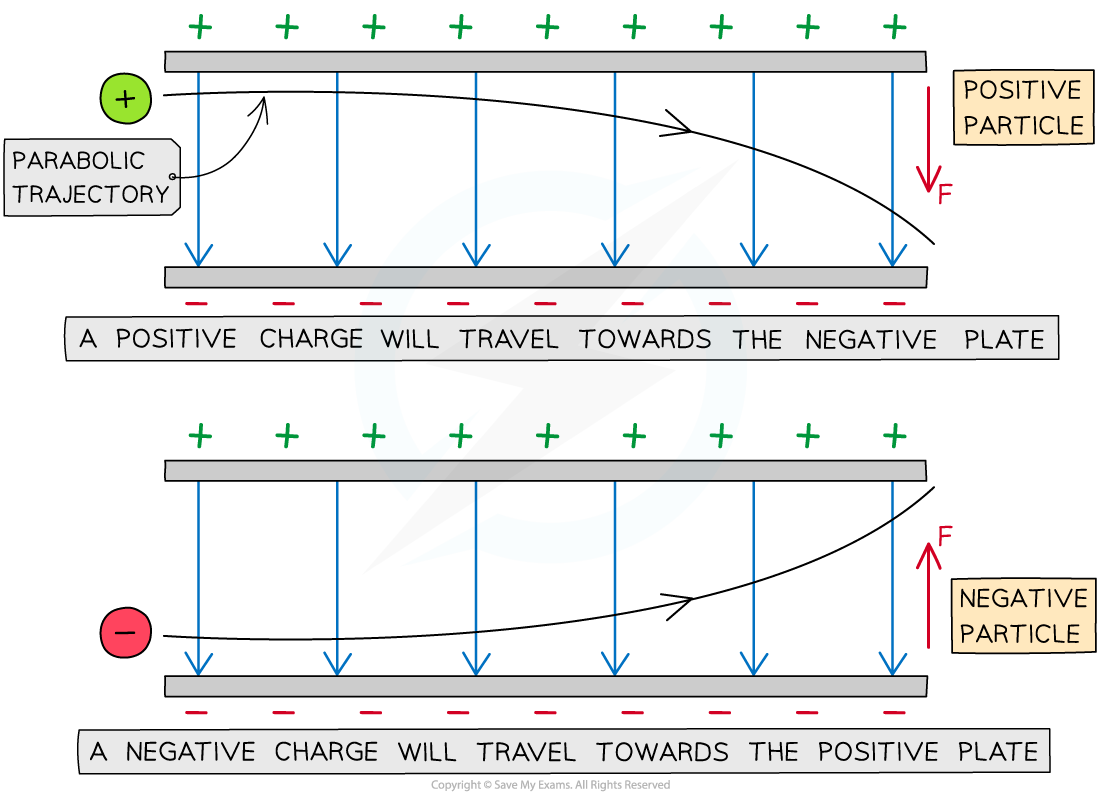# IB DP Physics: HL复习笔记10.2.8 Forces on Charges & Masses

### Forces on Charges & Masses

• The electric field strength equation can be rearranged for the force F on a charge Q in an electric field E:
•Where:
• F = electrostatic force on the charge (N)
• Q = charge (C)
• E = electric field strength (N C-1)
• The direction of the force is determined by the charge:
• If the charge is positive (+) the force is in the same direction as the E field
• If the charge is negative (-) the force is in the opposite direction to the E field
• The force on the charge will cause the charged particle to accelerate if its in the same direction as the E field, or decelerate if in the oppositeAn electric field strength E exerts a force F on a charge +Q in a uniform electric field

• Note: the force will always be parallel to the electric field lines

#### Motion of Charged Particles

• A charged particle in an electric field will experience a force on it that will cause it to move
• If a charged particle remains still in a uniform electric field, it will move parallel to the electric field lines (along or against the field lines depending on its charge)
• If a charged particle is in motion through a uniform electric field (e.g. between two charged parallel plates), it will experience a constant electric force and travel in a parabolic trajectoryThe parabolic path of charged particles in a uniform electric field

• The direction of the parabola will depend on the charge of the particle
• A positive charge will be deflected towards the negative plate
• A negative charge will be deflected towards the positive plate
• The force on the particle is the same at all points and is always in the same direction
• Note: an uncharged particle, such as a neutron experiences no force in an electric field and will therefore travel straight through the plates undeflected
• The amount of deflection depends on the following properties of the particles:
• Mass – the greater the mass, the smaller the deflection and vice versa
• Charge – the greater the magnitude of the charge of the particle, the greater the deflection and vice versa
• Speed – the greater the speed of the particle, the smaller the deflection and vice versa

#### Worked Example

A single proton travelling with a constant horizontal velocity enters a uniform electric field between two parallel charged plates.The diagram shows the path taken by the proton.Draw the path taken by a boron nucleus that enters the electric field at the same point and with the same velocity as the proton.Atomic number of boron = 5

Mass number of boron = 11

Step 1: Compare the charge of the boron nucleus to the proton

• Boron has 5 protons, meaning it has a charge 5 × greater than the proton
• The force on boron will therefore be 5 × greater than on the proton

Step 2: Compare the mass of the boron nucleus to the proton

• The boron nucleus has a mass of 11 nucleons meaning its mass is 11 × greater than the proton
• The boron nucleus will therefore be less deflected than the proton

Step 3: Draw the trajectory of the boron nucleus

• Since the mass comparison is much greater than the charge comparison, the boron nucleus will be much less deflected than the proton
• The nucleus is positively charged since the neutrons in the nucleus have no charge
• Therefore, the shape of the path will be the same as the proton